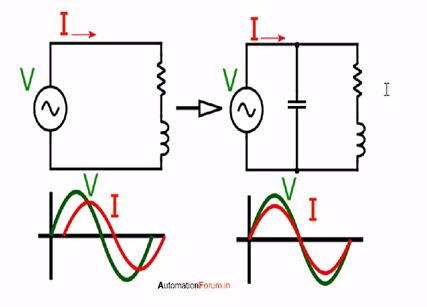# POWER FACTOR CORRECTION BY CAPACITORS

``````                POWER FACTOR CORRECTION BY CAPACITORS
``````

The power factor in an electrical system is the ratio of the real power that is used to do the work and the apparent power which is supplied to the circuit the value of the power factor ranges from 0 to 1. If we have a better power factor it could result in getting maximum current carrying capability power loss will be reduced and improved voltage to the equipment power factor can be improved by adding PF correction capacitors to the electrical system if the power factor is low then it draws higher internal current and the heat generated will be high and it will reduce the equipment life, the output voltage may be decreased due to the increased reactive load PhasecapTM capacitors are designed for AC applications phasecap capacitors are used widely because of its compact design high stability of capacitance improving energy quality and its service life is more than 10 000 h power factor is a measure of how effectively we use electricity In an electric utility company “unity power factor” is their main goal if the power factor is less than 1 they need to supply more current to the user than the given amount by doing that it cause more line losses.Power factor is defined as True power / Apparent power or watts / voltamperes

Voltage and current are sinusoidal true power = VI cos φ Apparent power is VI Power factor = true power / Apparent power
= VI cos φ / VI = cos φ

In an inductive series circuit where current lags voltage, the power factor is called as lagging power factor similarly in a capacitive series circuit where the current leads the voltage the power factor is called as leading power factor

POWER FACTOR CORRECTION There will be reactive current and active current in inductive devices such as motor or transformers load generators so we can use phasecapTM MKK capacitors to prevent most of this reactive current from reaching power generation or transmission system these capacitors are developed for power factor correction

BENEFITS OF POWER FACTOR CORRECTION • Effective utilization of electrical power • Reduced conduction losses in the cable due to less reactive current • Due to the power factor improvement, there is no need for the increase in cable size • Transmission losses are reduced • Power quality is improved

1 Like